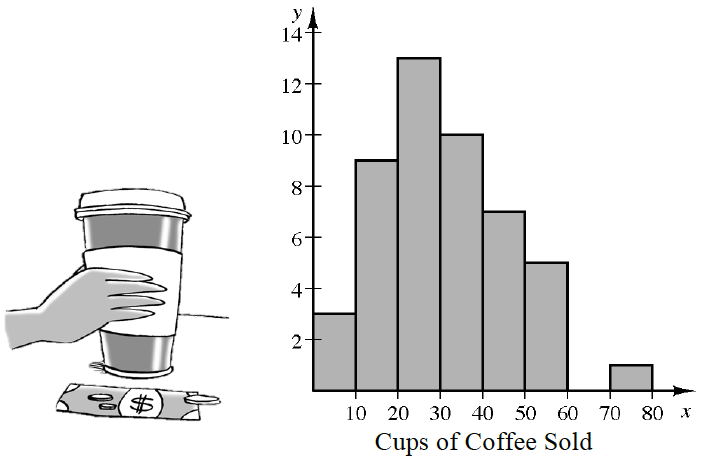### Home > CCA2 > Chapter 11 > Lesson 11.1.1 > Problem11-6

11-6.

In problem 9-8 Katelyn recorded the number of cups of coffee sold at her coffee shop each hour during a $48$-hour time period.

 Katelyn then sorted the data and made the following histogram:1. Katelyn decides to model her sales with a normal distribution, but Duncan thinks that this is not a good idea. What advice would you give Katelyn about using a normal model?

The data should be symmetric, single-peaked, and bell-shaped. Is it?

2. Katelyn needs to sell more than $15$ cups per hour to make a profit. What percentile is $15$ cups per hour? Explain the percentile to Katelyn in terms of profits.

Look at the data. How many data points sold less than $15$ cups of coffee?
Compare this to the total number of data points.

3. Duncan thinks Katelyn should expand her business. He estimates that if they hire another server and add more tables and equipment, they will need to sell $48$ cups per hour to make a profit. What percentile does $48$ cups sold represent? Use the percentile to explain to Duncan whether or not expansion is a good idea.

Use the same method as you did in part (b).
Note that this time it may be easier to count data points that sold more than $48$ cups.

 Cups of Coffee Sold $1$ $22$ $37$ $5$ $22$ $38$ $7$ $23$ $38$ $12$ $24$ $40$ $12$ $25$ $41$ $12$ $26$ $41$ $12$ $26$ $42$ $12$ $29$ $47$ $14$ $29$ $47$ $16$ $30$ $49$ $17$ $30$ $51$ $19$ $31$ $52$ $20$ $32$ $55$ $21$ $33$ $55$ $21$ $35$ $59$ $22$ $36$ $76$ checksum $1444$